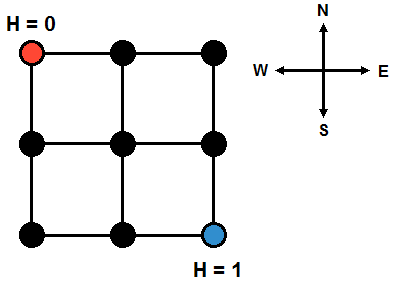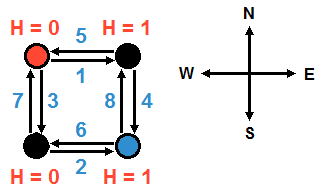시간 제한 메모리 제한 제출 정답 맞은 사람 정답 비율
1 초 512 MB 3 2 2 66.667%

문제

City YT is a well-designed city. The main roads that run east-west and north-south divide the city into n×n regions. Simply put, we can view city YT as a square with each region also being a square. From this, we know that city YT contains (n + 1)×(n + 1) intersections and 2n×(n+ 1) bidirectional streets (simply referred to as streets). Each street connects a pair of intersections on a main road. The diagram below depicts a map of city YT (n = 2) divided into 2×2 regions, including 3×3 intersections and 12 streets.Little Z is the mayor of this city. Using statistical information, he has obtained the bidirectional flow rate of people in each street during rush hours. For the duration of each rush hour, he will know the number of people going in a specific direction on a specific street. Each intersection also possesses an altitude, which is an issue that citizens of city YT find very stressful. For a person to climb up to an altitude of h will require him to exhaust h units of energy. If going downhill, no energy will be required. When traveling along a street, if the difference between the destination altitude and the initial altitude is h (note that h can be negative), then a person will consume max{0, h} units of energy when traveling along the street (here, max{ab} represents the largest of two numbers a and b).

Little Z also measured the altitude of the intersection at the north-west corner of city to be 0, and the altitude of the intersection at the south-east corner to be 1 (as depicted in the above diagram). However, there is no way to determine the altitudes of other intersections. Little Z would like to know in the most ideal situation (where you can freely assume altitudes for any intersection), what is the minimum total energy consumed by people climbing the slopes during rush hour?

입력

The first line of input consists of a single integer n, the meaning of which is described above.

For the following 4n(n + 1) lines, each line contains a nonnegative integer representing the number of people (i.e. the flow rate) passing through a particular street in a particular direction during rush hour.

The input order is: n(n + 1) numbers representing the flow rates of people going from west to east, followed by n(n + 1) numbers representing the flow rates of people going from north to south, followed by n(n + 1) numbers representing the flow rates of people going from east to west, finally followed by n(n + 1) numbers representing the flow rates of people going from south to north. For each directional flow rate, the input order will be from north to south according to initial locations. If the north-south position of the initial locations are the same, then the input order will be from west to east (see sample below).

출력

Output a single number, the minimum possible total energy consumption of all people traveling during rush hour if the altitudes were ideal. Round the answer (half-up) to the nearest integers.

제한

1 ≤ n ≤ 500, and 0 ≤ flow rate ≤ 1,000,000. Also, all flow rates will be integers.

1
1
2
3
4
5
6
7
8

3

힌트